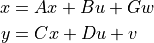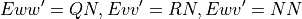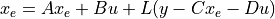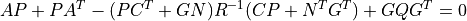# control.lqe¶

control.lqe(A, G, C, QN, RN[, NN])[source]

Linear quadratic estimator design (Kalman filter) for continuous-time systems. Given the systemwith unbiased process noise w and measurement noise v with covariancesThe lqe() function computes the observer gain matrix L such that the stationary (non-time-varying) Kalman filterproduces a state estimate x_e that minimizes the expected squared error using the sensor measurements y. The noise cross-correlation NN is set to zero when omitted.

The function can be called with either 3, 4, 5, or 6 arguments:

• `L, P, E = lqe(sys, QN, RN)`

• `L, P, E = lqe(sys, QN, RN, NN)`

• `L, P, E = lqe(A, G, C, QN, RN)`

• `L, P, E = lqe(A, G, C, QN, RN, NN)`

where sys is an LTI object, and A, G, C, QN, RN, and NN are 2D arrays or matrices of appropriate dimension.

Parameters
• A (2D array_like) – Dynamics, process noise (disturbance), and output matrices

• G (2D array_like) – Dynamics, process noise (disturbance), and output matrices

• C (2D array_like) – Dynamics, process noise (disturbance), and output matrices

• sys (LTI (StateSpace or TransferFunction)) – Linear I/O system, with the process noise input taken as the system input.

• QN (2D array_like) – Process and sensor noise covariance matrices

• RN (2D array_like) – Process and sensor noise covariance matrices

• NN (2D array, optional) – Cross covariance matrix. Not currently implemented.

• method (str, optional) – Set the method used for computing the result. Current methods are ‘slycot’ and ‘scipy’. If set to None (default), try ‘slycot’ first and then ‘scipy’.

Returns

• L (2D array (or matrix)) – Kalman estimator gain

• P (2D array (or matrix)) – Solution to Riccati equation• E (1D array) – Eigenvalues of estimator poles eig(A - L C)

Notes

1. If the first argument is an LTI object, then this object will be used to define the dynamics, noise and output matrices. Furthermore, if the LTI object corresponds to a discrete time system, the `dlqe()` function will be called.

2. The return type for 2D arrays depends on the default class set for state space operations. See `use_numpy_matrix()`.

Examples

```>>> L, P, E = lqe(A, G, C, QN, RN)
>>> L, P, E = lqe(A, G, C, Q, RN, NN)
```